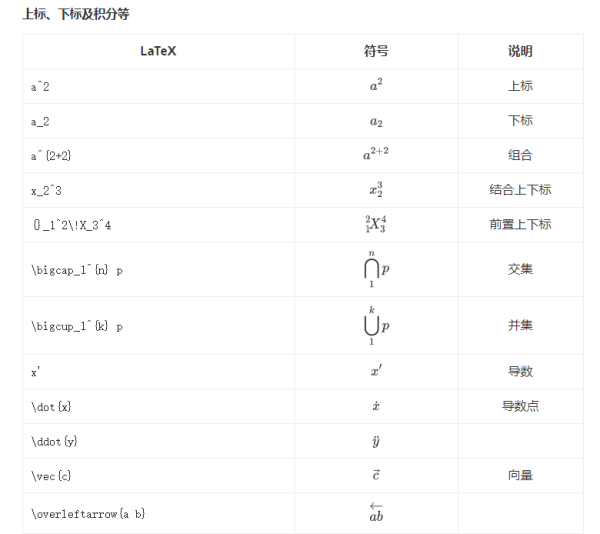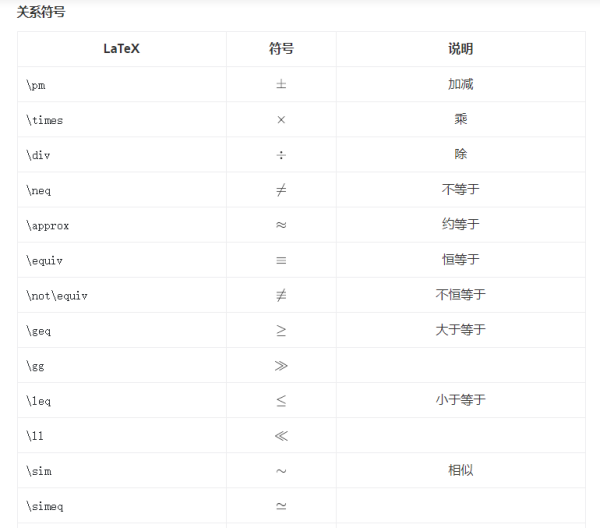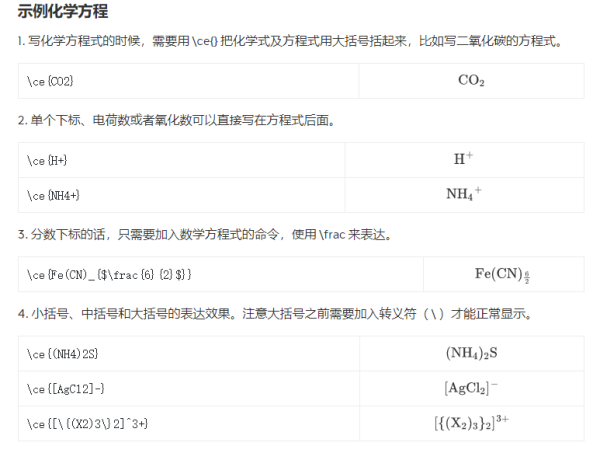# 你不知道的Xmind桌面版方程支持命令介绍

Xmind

Xmind作为一款头脑风暴类软件，由于它功能强大且全面，软件易于上手却上限很高，可支持企业的一些高级需求。此外，在Xmind的2020版本起，新增了公式插入功能，用户可以通过MathJax开源库，输入 LaTeX 数学命令，实时转化为数学公式。

Xmind桌面版的方程支持哪些命令

Xmind桌面版方程支持的命令一 上下标及积分a^2，上标;

a_2，下标;

a^{2+2}，组合;

x_2^3，结合上下标;

{}_1^2\!X_3^4，前置上下标;

\bigcap_1^{n} p，交集;

\overbrace{1+2+\cdots+100}，上括号;

\underbrace{a+b+\cdots+z}，下括号;

\sum_{k=1}^N k^2，求和;

\lim_{n \to \infty}x_n，极限;

\int_{-N}^{N} e^x\, dx，积分;

\iint_{D}^{W} \, dx\,dy，双重积分;

\oint_{C} x^3\, dx + 4y^2\, dy，曲面积分。

Xmind桌面版方程支持的命令二 关系符号\pm，加减;

\times，乘;

\div，除;

\neq，不等于;

\approx，约等于;

\equiv，恒等于;

\propto，正比于;

\perp，垂直。

Xmind桌面版方程支持的命令三 化学方程式\ce{CO2}，二氧化碳;

\ce{H+}，氢离子;

\ce{NH4+}，铵根离子;

\ce{CH3-CHO}，甲醛;

\ce{CH2=CH2}，乙烯;

\ce{CH#CH}，乙炔。

Xmind桌面版如何注册和登录

Xmind的操作指南

Xmind导图怎么导出成图片

• 热门标签
• 热门搜索
• 精选软件

• 每周热文
• 每月热文

## 你不知道的Xmind桌面版方程支持命令介绍Xmind